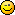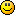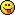## Discuss Scratch

### Power block

This makes me think, they should add this:
`(() √ ()) // category=operators`

Last edited by savaka (Dec. 26, 2013 23:17:03)

### Power block

Support for a new ((x) ^ (y)) block.
1. it is simpler than other solutions
2. it is faster than other solutions
3. at least in the form ((x) ^ (2)) it will be used a lot
4. young scratchers (below 10) might not know what ^is, but the same holds for log, sin, cos, mod, … - at least the code of others becomes easier to read

`((x) ^ (y))`
(but in green)

Last edited by frodewin (Dec. 23, 2013 18:06:47)

### Power block

You mean like
`(() ^ ()) // category=operators`
Anyway…

savaka wrote:

This makes me think, they should add this:
`(() √ ()) // category=operators`
`([sqrt v] of ())`
Also, that block would make you input the answer. It would be a boolean block, not a reporter block.

Last edited by mariobros406 (Dec. 23, 2013 21:02:41)

I only check in to Scratch from time to time to see what others have been up to. I don't really post anything anymore. I'll occasionally post a question in the AT forum if I can't find an answer anywhere else.

### Power block

mariobros406 wrote:

You mean like
`(() ^ ()) // category=operators`
Anyway…

savaka wrote:

This makes me think, they should add this:
`(() √ ()) // category=operators`
`([sqrt v] of ())`
Also, that block would make you input the answer. It would be a boolean block, not a reporter block.
No, a
` (() √ ()) // category=operators `
block would be a reporter. The first number would be the index or degree while the second would be the radicand. When we say “square root” that means it has an index of 2, since squares are 2 dimensional. If a number was cubed (having an index of 3) you find what number multiplies by itself 3 times to get that number, rather than the 2 from a square root.

10-year Scratch veteran. Fight me.

### Power block

mariobros406 wrote:

You mean like
`(() ^ ()) // category=operators`
Anyway…

savaka wrote:

This makes me think, they should add this:
`(() √ ()) // category=operators`
`([sqrt v] of ())`
Also, that block would make you input the answer. It would be a boolean block, not a reporter block.
You can set categories? Cool! Changing…

### Power block

savaka wrote:

mariobros406 wrote:

You mean like
`(() ^ ()) // category=operators`
Anyway…

savaka wrote:

This makes me think, they should add this:
`(() √ ()) // category=operators`
`([sqrt v] of ())`
Also, that block would make you input the answer. It would be a boolean block, not a reporter block.
You can set categories? Cool! Changing…
Yup. But don't use it too often…it's top secret!

I only check in to Scratch from time to time to see what others have been up to. I don't really post anything anymore. I'll occasionally post a question in the AT forum if I can't find an answer anywhere else.

### Power block

sccar3 wrote:

mariobros406 wrote:

You mean like
`(() ^ ()) // category=operators`
Anyway…
No, a
` (() √ ()) // category=operators `
block would be a reporter. The first number would be the index or degree while the second would be the radicand. When we say “square root” that means it has an index of 2, since squares are 2 dimensional. If a number was cubed (having an index of 3) you find what number multiplies by itself 3 times to get that number, rather than the 2 from a square root.

Too easy workaround.
`(((1) / (amount)) ^ (other amount)) // category=operators`

mariobros406 wrote:

savaka wrote:

You can set categories? Cool! Changing…
Yup. But don't use it too often…it's top secret!
Not too top-secret when you tell everyone that asksLast edited by 1234abcdcba4321 (Dec. 26, 2013 19:31:11)

I'd highly appreciate it if you were to follow me. Don't forget to go to my profile.
All of my programming suggestions <— You should really get the suggestions move back to the suggestions forum!
Five hundred posts! I never expected to get up there… In only 2-3 months.
Does anyone know what a signature is? I mean, I've already seen 6-7 people get confused.

### Power block

mariobros406 wrote:

savaka wrote:

mariobros406 wrote:

You mean like
`(() ^ ()) // category=operators`
Anyway…

savaka wrote:

This makes me think, they should add this:
`(() √ ()) // category=operators`
`([sqrt v] of ())`
Also, that block would make you input the answer. It would be a boolean block, not a reporter block.
You can set categories? Cool! Changing…
Yup. But don't use it too often…it's top secret!

1234abcdcba4321 wrote:

sccar3 wrote:

mariobros406 wrote:

You mean like
`(() ^ ()) // category=operators`
Anyway…
No, a
` (() √ ()) // category=operators `
block would be a reporter. The first number would be the index or degree while the second would be the radicand. When we say “square root” that means it has an index of 2, since squares are 2 dimensional. If a number was cubed (having an index of 3) you find what number multiplies by itself 3 times to get that number, rather than the 2 from a square root.

Too easy workaround.
`(((1) / (amount)) ^ (other amount)) // category=operators`

mariobros406 wrote:

savaka wrote:

You can set categories? Cool! Changing…
Yup. But don't use it too often…it's top secret!
Not too top-secret when you tell everyone that asks1. You can just see the code by rolling over any of the blocks on the first menu.2. You're right, the workaround is easy, but no everyone knows that.

### Power block

1234abcdcba4321 wrote:

sccar3 wrote:

mariobros406 wrote:

You mean like
`(() ^ ()) // category=operators`
Anyway…
No, a
` (() √ ()) // category=operators `
block would be a reporter. The first number would be the index or degree while the second would be the radicand. When we say “square root” that means it has an index of 2, since squares are 2 dimensional. If a number was cubed (having an index of 3) you find what number multiplies by itself 3 times to get that number, rather than the 2 from a square root.

Too easy workaround.
`(((1) / (amount)) ^ (other amount)) // category=operators`

Not exactly, the workaround for the n-th square root of x would be

`((x) ^ ((1) / (amount))) // category=operators`

Therefore I don't think we would need an n-th square root block, but a power block would be useful.

### Power block

1234abcdcba4321 wrote:

sccar3 wrote:

mariobros406 wrote:

You mean like
`(() ^ ()) // category=operators`
Anyway…
No, a
` (() √ ()) // category=operators `
block would be a reporter. The first number would be the index or degree while the second would be the radicand. When we say “square root” that means it has an index of 2, since squares are 2 dimensional. If a number was cubed (having an index of 3) you find what number multiplies by itself 3 times to get that number, rather than the 2 from a square root.

Too easy workaround.
`(((1) / (amount)) ^ (other amount)) // category=operators`

mariobros406 wrote:

savaka wrote:

You can set categories? Cool! Changing…
Yup. But don't use it too often…it's top secret!
Not too top-secret when you tell everyone that asksI only check in to Scratch from time to time to see what others have been up to. I don't really post anything anymore. I'll occasionally post a question in the AT forum if I can't find an answer anywhere else.

### Power block

frodewin wrote:

1234abcdcba4321 wrote:

sccar3 wrote:

mariobros406 wrote:

You mean like
`(() ^ ()) // category=operators`
Anyway…
No, a
` (() √ ()) // category=operators `
block would be a reporter. The first number would be the index or degree while the second would be the radicand. When we say “square root” that means it has an index of 2, since squares are 2 dimensional. If a number was cubed (having an index of 3) you find what number multiplies by itself 3 times to get that number, rather than the 2 from a square root.

Too easy workaround.
`(((1) / (amount)) ^ (other amount)) // category=operators`

Not exactly, the workaround for the n-th square root of x would be

`((x) ^ ((1) / (amount))) // category=operators`

Therefore I don't think we would need an n-th square root block, but a power block would be useful.
Square root is only 2√. n√ is not also a square root.

### Power block

Support, but I think it should be
`(() ** ())//category=operators`

### Power block

davidkt wrote:

Support, but I think it should be
`(() ** ())//category=operators`
I don't get it.
`(() ^ ()) // category=operators`
is the actual symbol for power and people would understand it.

### Power block

firedrake969_test wrote:

The thing is that you can't do negative numbers with logs.
OK, so here's my definitive x^y custom block…
`define result = (x) ^ (y)if <(y) = > then  set [result v] to  // yes, we also include 0^0=1  :Oelse  if <(x) = > then    set [result v] to   // 0^y=0 for any y except zero (see above)  else    set [result v] to ([e^ v] of ((y)*([ln v] of ([abs v] of (x)))) // no negative x for now    if <(x) < > then  // now deal with power of negative number      if <(round(y)) = (y)> then // we can do integer powers of negative numbers        if <((y) mod (2)) = > then          set [result v] to ((0) - (result)) // odd powers will be negative        end      else        set [result v] to [NaN] // but let's not go there at this stage...      end    end    if <<(y) > > and <<(round(x)) = (x)> and <(round(y)) = (y)>>> then      set [result v] to (round (result)) // ensure we get exactly an integer if both x & y were ints    end  endend`
That should cover everything but non-integer powers of negative numbers (which gets a bit hairy…), and it makes sure that integer raised to integer gives exactly an integer (in case you assume you'll get an integer, perhaps because you check for equality with an integer at some point).

Hope I've got that all right!

Have you put this is a project?

### Power block

scubajerry wrote:

Have you put this is a project?
OK, here it is:http://scratch.mit.edu/projects/16871883
(Plus a couple of extra tweaks)Last edited by DadOfMrLog (Jan. 22, 2014 19:15:34)

### Power block

frodewin wrote:

Support for a new ((x) ^ (y)) block.
1. it is simpler than other solutions
2. it is faster than other solutions
3. at least in the form ((x) ^ (2)) it will be used a lot
4. young scratchers (below 10) might not know what ^is, but the same holds for log, sin, cos, mod, … - at least the code of others becomes easier to read

`((x) ^ (y))`
(but in green)
I'm 7 and know that..You won't be unknown anymore
My browser / operating system: Windows 7, Chrome 32.XX.XXXX.XX, Flash 12.0 (release 0)
My alternative browser / operating system: OS X 10.9.5, Safari 7, Flash 15.0 (release 0)
Reached 500+ on April 8, 2015

### Power block

I'm sorry if I'm necroposting here, but I support.### Power block

You could just use a repeat block…

Last edited by Haiming (July 9, 2014 13:24:29)

### Power block

davidkt wrote:

Support, but I think it should be
`(() ** ())//category=operators`
Now I get it. ** is how they do it in Python. But this isn't Python. It's Scratch. And people know it as ^, because that's the math symbol.

### Power block

`define x to the power of y`